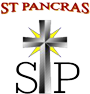# Maths

Bola and Italic milestones have been taught

 NUMBER, PLACE VALUE & ROUNDING Y2.27 I count in steps of 3 Y2.28 I count forwards and backwards in tens from any number Y2.29 I know the place value of each digit in a 2-digit number Y2.30 I estimate quantities Y2.31 I order numbers from 0 to 100 Y2.32 I use the < > signs Y2.33 I read and write numbers to at least 100 in numerals and words ADDITION AND SUBTRACTION Y2.34 I solve one step problems with + and - Y2.35 I know number facts to 100 Y2.36 I add and subtract 2 digit numbers to 20 Y2.37 I show that addition can be done in any order and subtraction cannot MULTIPICATION AND DIVISION Y2.38 I instantly recall and use x facts for the 2, 5 and 10 x tables Y2.39 I instantly recall and use ÷ facts for the 2, 5 and 10 x tables Y2.40 I know odd and even numbers Y2.41 I know that x and ÷ are the inverse of one another Y2.42 I know that multiplying two numbers can be done in any order Y2.43 I solve one step problems using multiplication and division FRACTIONS Y2.44 I find, name and write fractions of a length Y2.45 I find, name and write fractions of a shape (1/2, 1/3, 1/4) Y2.46 I find, name and write fractions of a set of objects (1/2, 1/3, 1/4) Y2.47 I find, name and write fractions of a number (1/2, 1/3, 1/4) Y2.48 I write fractions and recognise some equivalences MEASURES Y2.49 I use standard unit to estimate and measure accurately Y2.50 I use some equipment to measure accurately Y2.51 I compare and order length, mass, volume and capacity Y2.52 I use symbols for pounds and pence Y2.53 I solve money problems Y2.54 I compare and sequence intervals of time Y2.55 I solve problems involving measures Y2.56 I know and use words relating to dates – days, weeks and months GEOMETRY Y2.57 I know the properties of 2D shapes Y2.58 I identify lines of symmetry in 2D shapes Y2.59 I know the properties of 3D shapes Y2.60 I compare and sort 2D and 3D shapes DATA Y2.61 I interpret and construct pictograms Y2.62 I interpret and construct tally charts Y2.63 I interpret and construct block diagrams Y2.64 I interpret and construct tables Y2.65 I ask and answer questions about totalling

White Rose Maths Yearly overview

We follow White Rose as the basis of our maths curriculum, with the yearly overview of topics attached below.